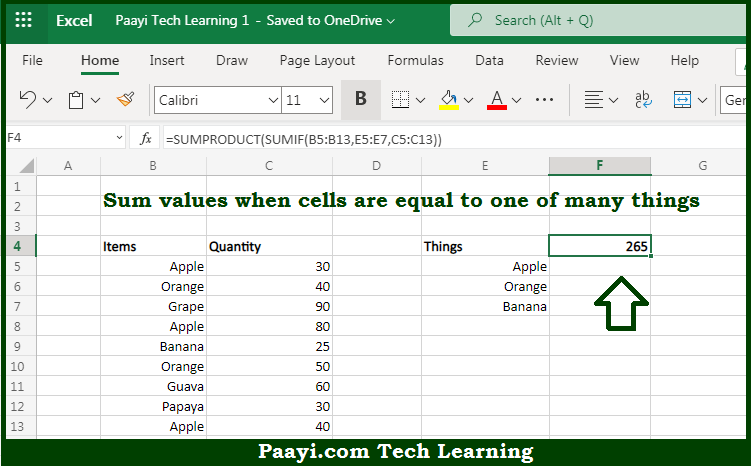# Learn How to SUM If Equal to One of Many Things in Microsoft Excel

Written by | 0 Comments | 455 Views

In this article, you will learn how to SUM various things in Microsoft Excel using a single/combination(s) of functions. You will also know how to SUM If Equal to One of Many Things and see the generic formula.

SUM If Equal to One of Many Things in Microsoft Excel

The main purpose of this formula is to sum values when cells are equal to one of many things. Here we will learn how to sum equal to one of many things in the workbook in Microsoft Excel. That implies, with the help of a formula based on the SUMIF and SUMPRODUCT function you can able to sum values when cells are equal to one of many things. So, with the help of this formula, you can able to sum equal to one of many things in the workbook in Microsoft Excel.

General Formula to SUM If Equal to One of Many Things

=SUMPRODUCT(SUMIF(range,things,values))

The Explanation for the SUM If Equal to One of Many ThingsSo we know that with the help of the given formula above you can able to sum values when cells are equal to one of many things. Here we will learn how to sum equal to one of many things in the workbook in Microsoft Excel. As we know that the SUMIF function supports  logical operators efficiently, which are -  "=",">",">=", etc. So you can able to use these operators as per your criteria. It should be noted that as per our example above the SUMIF function all three values in the named range things E column as criteria, then use the SUMPRODUCT function is used to acquire total. So, with the help of this formula, you can able to sum equal to one of many things in the workbook in Microsoft Excel.